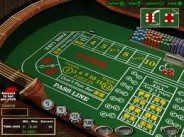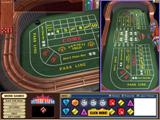ReadyBetGo! HomeCraps HomeCraps StrategyDice ControlCraps Books
Club USA Casino CrapsClub USA Casino has good craps action at their site. They offer private, public and reserved tables. The minimum bet is \$1 and the max is \$500. The casino offers behind the line odds. Nice graphics and sound add up to a satisfying craps experience. Good shooting.
Play Now at Club USA Casino!
Intercasino CrapsFeel like playing at a funky craps table where you shoot the dice just like in Vegas? That's what Intercasino craps has to offer. The software gives you two choices, you can watch the dice or throw them. The throwing action may be just an illusion, but it's a good one where you feel you can put some action on the bones. Come on seven!

# Remembering Craps Pass Line Odds

When you play craps, you probably know the payoffs for most numbers on place bets. For example, the odds on the six and eight are 7:6 (bet \$6, get \$7), the odds on the five and nine are 7:5Larry Edell has been the editor of 'The Crapshooter Newsletter' since 1994. He has published nine books and over two hundred different articles in magazines such as 'Casino Player', 'Gaming Today', 'Mid West Players 'and 'Gambling Times'.  Larry's website is www.thecrapshooter.com  (bet \$5, get \$7) and the odds on the four and ten are 9:5 (bet \$5, get \$9). But, when you want to add odds to your pass or come bets, the payoff is different. This is because they are true odds, which means that the casino has no advantage on these odds bets.

The odds for the numbers six and eight are the same, 6:5. The odds for both the five and nine are 3:2 and the odds for the four and ten are 2:1. If you just subtract one from the smaller of these numbers and relate it to six (the number of times a seven rolls) you get the true odds.

For example, to get the true odds for the four or ten, just subtract one from four (four is smaller than ten) to get three. So the true odds of the four or ten are 6:3, or 2:1.

To get the true odds for the six or eight, subtract one from six (six is smaller than eight) to get five. So the true odds for the six or eight are 6:5.

And finally, to get the true odds for the five or nine, subtract one from five (five is smaller than nine) to get four, so the odds of the five or nine are 6:4, or 3:2.

Here are some examples with some typical added odds bets.

If you have \$10 on the come or pass line and your point is a five or nine, and you want to add some odds, just subtract one from five to get four. The true odds then is 6:4, or 3:2, so if you bet \$20, you'll win \$30. For double odds you can add \$20 odds to your \$10 flat bet and win \$10 from the flat portion and \$30 from the odds portion, for a total of \$40.

If the point is four or ten, subtract one from four to get three. The true odds then are 6:3, or 2:1, so if you bet \$20, you'll win \$40. For double odds, you can add \$20 to your flat \$10 bet and win \$10 on the flat portion and \$40 to the odds portion, for a total of \$50.

The six or eight is a little different. If you subtract one from six you get five, which means the true odds are 6:5. If you have a flat bet of \$10, you would be able to take \$20 in odds, (on a 2X odds table) however, this would mean a \$24 odds payout, which is awkward for most casinos. So, to even things up, most casinos let you place \$25 in odds behind the 6 or 8, (which really gives you 2.5X odds instead 2X), giving you \$10 for the flat portion and an even \$30 for the odds portion, for a total win of \$40.

This method works on don't-come and don't-pass bets as well, except the odds are reversed. This is because on the don't side you have the advantage rather than the house, so you have to bet more to get less.

If your don't-pass or don't-come flat bet is \$10 and the point is five or nine, subtract one from five to get four, giving you odds of 4:6 (instead of 6:4), or 2:3. This means you have to bet \$30 (on a 2X table) in odds to get a \$20 payout, winning you a total of \$30 (\$10 flat + \$20 odds).

If your don't number is four or ten, subtract one from four to get three, so the odds are 3:6, or 1:2. You would have to bet \$40 in odds to get a \$20 payout, winning you a total of \$30 (\$10 flat + \$20 odds).

For the don't six or eight, subtract one from six to get five, so the odds are 5:6. You would have to bet \$24 in odds to get a \$20 payout, winning you a total of \$30 (\$10 flat + \$20 odds).

The next time you play craps, remember this simple method to calculate the true odds. This way, you'll know what odds to give the dealers, and what the correct payoff should be.